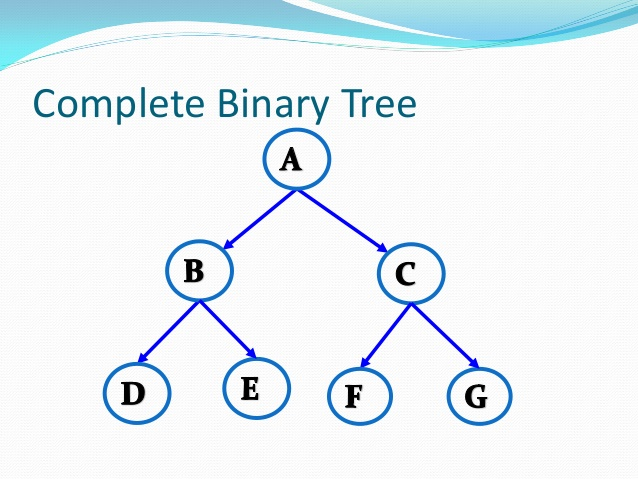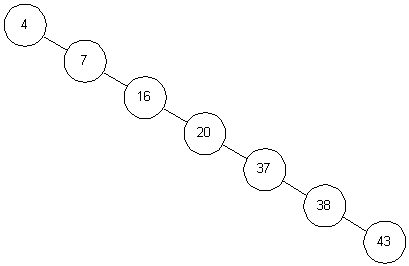# Answered! The time taken to find a node in a binary search tree is a function of the height of the tree. For a binary tree with N…

The time taken to find a node in a binary search tree is a function of the height of the tree. For a binary tree with N nodes, what are the upper and lower bounds on the height of the tree and why? [4 marks]

-A binary tree is a tree in which each node can have at most 2 child nodes.
-In binary search tree the lower bound in terms of height is given by complete BST and the unbalanced tree gives the upper bound to the height.

Don't use plagiarized sources. Get Your Custom Essay on
Answered! The time taken to find a node in a binary search tree is a function of the height of the tree. For a binary tree with N…
GET AN ESSAY WRITTEN FOR YOU FROM AS LOW AS \$13/PAGE-A Complete binary is one whose all levels except the last one is completely filled and on the last level all the child nodes are all to the left side and the unbalanced is the one that has difference in the depth of the two sub trees of every node by more than 1.
-So keeping this in mind, we can see that a BST can have the upper bound on height if it is a unbalanced BST, where nodes are either completely on the left or on the right side of the tree. In this arrangement each individual node is contributing to the height of tree. So if we have a tree of N nodes then the upper bound to height is N.
-Whereas if the tree is balanced then the height attained is the lower bound for BST. With each new level 2levelno nodes are contributed to the new level. So with this arrangement least height is attained with the number of nodes. So the lower bound of height for BST= log2(N+1).

log2(N+1) <= Height of BST <= N